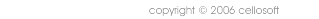# SQL Schema Type System

The SQL Schema type system is the subset of SQL Schema that allows you to define data types.

A type in SQL Schema has the following:

• Some form of data storage
• Constraints to determine validate data input
• Methods for working with the type (editing, displaying, analyzing)
• Values that can be assigned to the type (constants or SQL functions)
• A base type to inherit all of the above from

The type system provides an XML structure to define all of the above, while leaving the actual implementation of methods, sql types, and sql functions to the program.

## Syntax

Types are defined in XML and look like the following:

```<type name="Username" base="String" size="15">  <constrain type="length" min="3" />  <constrain type="ereg" value="^[a-zA-Z0-9]+\$"/></type><type name="Password" base="String" size="15">  <constrain type="length" min="6" />  <constrain type="ereg" value="^[a-zA-Z0-9]+\$"/></type><type name="Email" base="String" size="255">  <constrain type="length" min="5"/>  <constrain type="ereg" value="^.+@.+\$"/></type> <type name="Title" base="String">
<default value="No title"/>
</type><type name="Message" base="String" size="^2" /><type name="Date" base="Timestamp">  <value name="default"/>  <value name="yesterday"/></type>

<type name="Status" base="Enum">